# C# Decision Making (if, if-else, if-else-if ladder, nested if, switch, nested switch)

Decision Making in programming is similar to decision making in real life. In programming too, a certain block of code needs to be executed when some condition is fulfilled.
A programming language uses control statements to control the flow of execution of program based on certain conditions. These are used to cause the flow of execution to advance and branch based on changes to the state of a program.

The conditional statements of C#:

IF Statement
The if statement checks the given condition. If the condition evaluates to be true then the block of code/statemnts will execute otherwise not.
Syntax:

```if(condition)
{
//code to be executed
}  ```

Note: If the curly brackets { } are not used with if statements than the statement just next to it is only considered associated with the if statement.
Example:

```if (condition)
statement 1;
statement 2;```

In this example, only statement 1 is considered to be associated with the if statement.

Flowchart: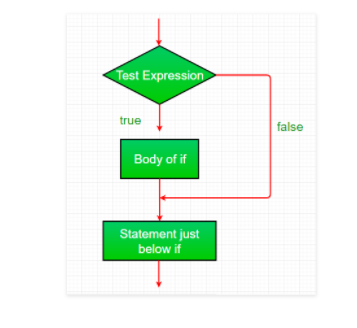Example:

 `// C# program to illustrate if statement ` `using` `System; ` ` `  `public` `class` `GFG { ` ` `  `    ``public` `static` `void` `Main(``string``[] args) ` `    ``{ ` `        ``string` `name = ``"Geek"``; ` `        ``if` `(name == ``"Geek"``) { ` `            ``Console.WriteLine(``"GeeksForGeeks"``); ` `        ``} ` `    ``} ` `} `

Output:

`GeeksForGeeks`

IF – else Statement
The if statement evaluates the code if the condition is true but what if the condition is not true, here comes the else statement. It tells the code what to do when the if condition is false.

Syntax:

```    if(condition)
{
// code if condition is true
}
else
{
// code if condition is false
}
```

Flowchart: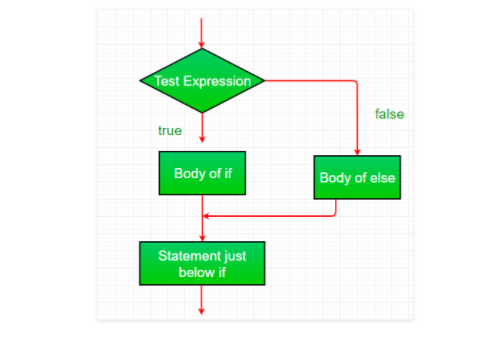Example:

 `// C# program to illustrate  ` `// if-else statement ` `using` `System; ` ` `  `public` `class` `GFG { ` ` `  `    ``public` `static` `void` `Main(``string``[] args) ` `    ``{ ` `        ``string` `name = ``"Geek"``; ` `        ``if` `(name == ``"Geeks"``) { ` `            ``Console.WriteLine(``"GeeksForGeeksr"``); ` `        ``} ` `        ``else` `{ ` `            ``Console.WriteLine(``"Geeks"``); ` `        ``} ` `    ``} ` `} `

Output:

`Geeks`

If – else – if ladder Statement
The if-else-if ladder statement executes one condition from multiple statements. The execution starts from top and checked for each if condition. The statement of if block will be executed which evaluates to be true. If none of the if condition evaluates to be true then the last else block is evaluated.

Syntax:

```        if(condition1)
{
// code to be executed if condition1 is true
}
else if(condition2)
{
// code to be executed if condition2 is true
}
else if(condition3)
{
// code to be executed if condition3 is true
}
...
else
{
// code to be executed if all the conditions are false
}
```

Flowchart: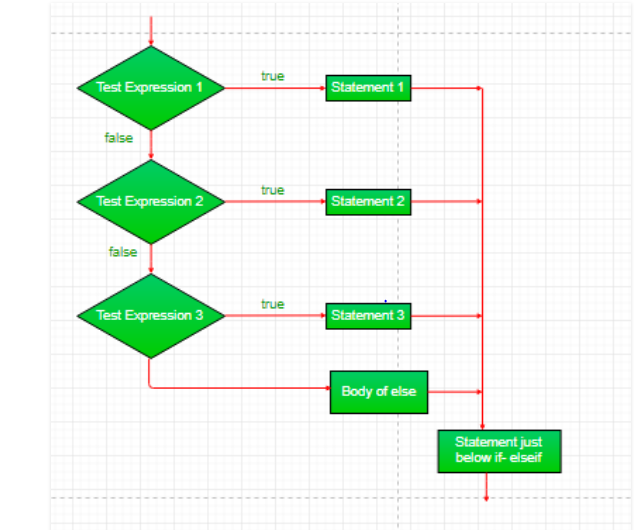Example:

 `// C# program to illustrate ` `// if-else-if ladder ` `using` `System; ` ` `  `class` `GFG { ` ` `  `    ``public` `static` `void` `Main(String[] args) ` `    ``{ ` `        ``int` `i = 20; ` ` `  `        ``if` `(i == 10) ` `            ``Console.WriteLine(``"i is 10"``); ` `        ``else` `if` `(i == 15) ` `            ``Console.WriteLine(``"i is 15"``); ` `        ``else` `if` `(i == 20) ` `            ``Console.WriteLine(``"i is 20"``); ` `        ``else` `            ``Console.WriteLine(``"i is not present"``); ` `    ``} ` `} `

Output:

`i is 20`

Nested – If Statement
if statement inside an if statement is known as nested if. if statement in this case is the target of another if or else statement. When more then one condition needs to be true and one of the condition is the sub-condition of parent condition, nested if can be used.

Syntax:

```        if (condition1)
{
// code to be executed
// if condition2 is true
if (condition2)
{
// code to be executed
// if condition2 is true
}
}
```

Flowchart: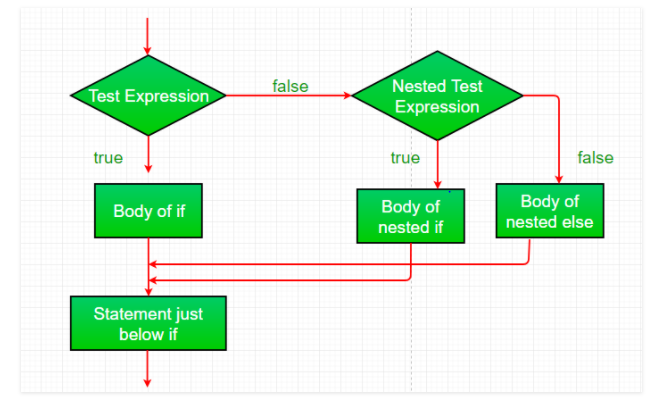Example:

 `// C# program to illustrate ` `// nested-if statement ` `using` `System; ` ` `  `class` `GFG { ` ` `  `    ``public` `static` `void` `Main(String[] args) ` `    ``{ ` `        ``int` `i = 10; ` ` `  `        ``if` `(i == 10) { ` ` `  `            ``// Nested - if statement ` `            ``// Will only be executed if statement ` `            ``// above it is true ` `            ``if` `(i < 12) ` `                ``Console.WriteLine(``"i is smaller than 12 too"``); ` `            ``else` `                ``Console.WriteLine(``"i is greater than 15"``); ` `        ``} ` `    ``} ` `} `

Output:

`i is smaller than 12 too`

Switch Statement
Switch statement is an alternative to long if-else-if ladders. The expression is checked for different cases and the one match is executed. break statement is used to move out of the switch. If the break is not used, the control will flow to all cases below it until break is found or switch comes to an end. There is default case (optional) at the end of switch, if none of the case matches then default case is executed.

Syntax:

```switch (expression)
{
case value1: // statement sequence
break;
case value2: // statement sequence
break;
.
.
.
case valueN: // statement sequence
break;
default: // default statement sequence
}
```

Flow Diagram of Switch – case :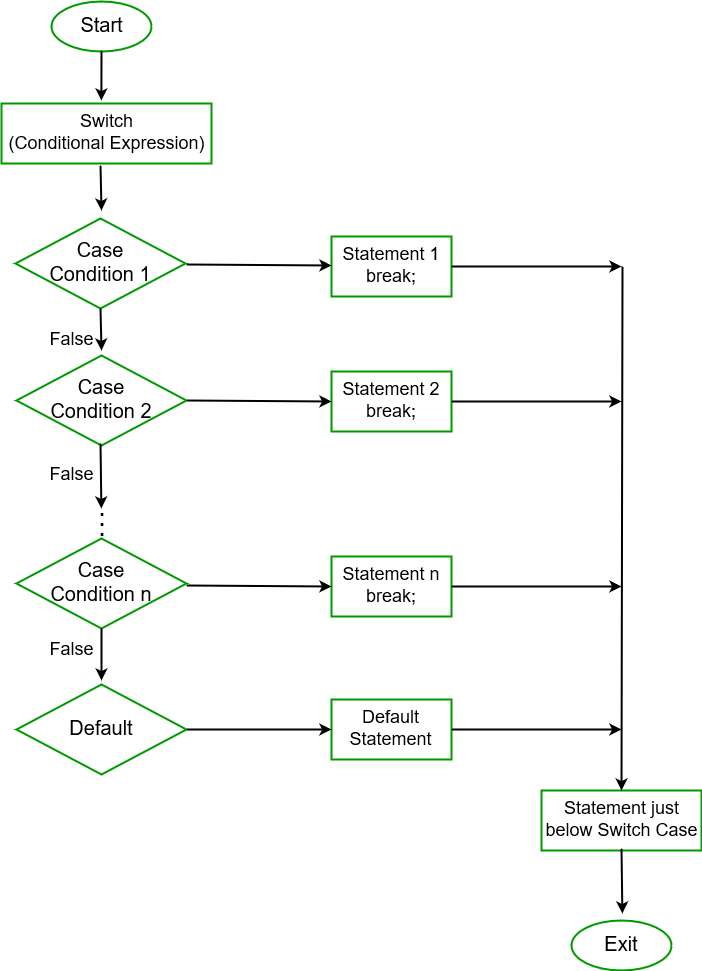Example:

 `// C# example for switch case ` `using` `System; ` ` `  `public` `class` `GFG  ` `{ ` `    ``public` `static` `void` `Main(String[] args) ` `    ``{ ` `        ``int` `number = 30; ` `        ``switch``(number) ` `        ``{ ` `        ``case` `10: Console.WriteLine(``"case 10"``); ` `                 ``break``; ` `        ``case` `20: Console.WriteLine(``"case 20"``); ` `                 ``break``; ` `        ``case` `30: Console.WriteLine(``"case 30"``); ` `                 ``break``; ` `        ``default``: Console.WriteLine(``"None matches"``);  ` `                 ``break``; ` `        ``} ` `    ``} ` `} `

Output:

`case 30`

Nested switch
Nested Switch case are allowed in C# . In this case, switch is present inside other switch case. Inner switch is present in one of the cases in parent switch.

Example:

 `// C# example for nested switch case ` `using` `System; ` ` `  `public` `class` `GFG  ` `{ ` `    ``public` `static` `void` `Main(String[] args) ` `    ``{ ` `        ``int` `j = 5; ` ` `  `        ``switch` `(j) ` `        ``{ ` `            ``case` `5: Console.WriteLine(5); ` `                    ``switch` `(j - 1) ` `                   ``{ ` `                    ``case` `4: Console.WriteLine(4); ` `                            ``switch` `(j - 2) ` `                          ``{ ` `                            ``case` `3: Console.WriteLine(3); ` `                                    ``break``; ` `                         ``} ` `                    ``break``; ` `                ``} ` `                ``break``; ` `            ``case` `10: Console.WriteLine(10); ` `                     ``break``; ` `            ``case` `15: Console.WriteLine(15); ` `                     ``break``; ` `            ``default``: Console.WriteLine(100); ` `                     ``break``; ` `        ``} ` ` `  `    ``} ` `} `

Output:

```5
4
3```

My Personal Notes arrow_drop_upCheck out this Author's contributed articles.

If you like GeeksforGeeks and would like to contribute, you can also write an article using contribute.geeksforgeeks.org or mail your article to contribute@geeksforgeeks.org. See your article appearing on the GeeksforGeeks main page and help other Geeks.

Please Improve this article if you find anything incorrect by clicking on the "Improve Article" button below.

Article Tags :

2

Please write to us at contribute@geeksforgeeks.org to report any issue with the above content.